## Wednesday, November 18, 2009

### Bertrand's Postulate (Theorem)

In today's blog, I will present a proof for Bertrand's Postulate which is really a theorem.

The content is taken primarily from an excellent Wikipedia article.

Lemma 1:

If n is greater than 5, then 2n/3 is greater than 2n

Proof:

(1) For n ≥ 5, 2n(2n-9) is greater than 0 since 2n ≥ 10 and 2n-9 ≥ 1.

(2) 2n(2n - 9) = 4n2 - 18n.

(3) Since 4n2 - 18n is greater than 0, 4n2 is greater than 18n.

(4) Dividing both sides by 9 gives us 4n2/9 is greater than 2n.

(5) Taking the square root of both sides gives us 2n/3 is greater than 2n

QED

Lemma 2:

Let p be a prime number that is greater than 2n

Let x be the highest nonnegative integer such that px divides 2n!/[(n!)(n!)]

then: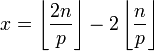Proof:

(1) We know that in general, the maximum power of p that divides n! is (see Theorem, here):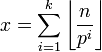(2) So the highest power of p that divides (2n)!/[(n!)(n!)] is: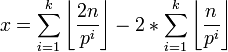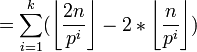(3) Since p is greater than 2n, it follows that p2 is greater than 2n. So we only need to consider the case where i=1.

(4) This leaves us with:QED

Lemma 3:

Bertrand's Posulate is true for n less than 2048

Proof:

Between any n less than 2048 and its double, one will find one of the following primes:

3, 5, 7, 13, 23, 43, 83, 163, 317, 631, 1259 and 2503

since:

n = 2 --> 3

n between 3 and 4 --> 5

n between 5 and 6 --> 7

n between 7 and 12 --> 13

n between 13 and 22 --> 23

n between 23 and 42 --> 43

n between 43 and 82 --> 83

n between 83 and 162 --> 163

n between 163 and 316 --> 317

n between 317 and 630 --> 631

n between 631 and 1258 --> 1259

n between 1259 and 2047 --> 2503

QED

Definition 1: R(p,n): maximum divisor of 2n!/(n!)(n!)

Let R(p,n) be the function that returns the maximum power of a given prime that divides 2n!/(n!)(n!)

For any prime p, pR(p,n) divides 2n!/(n!)(n!) but pR(p,n)+1 does not.

Example 1: R(3,5)

(2*5)!/(5!)(5!) = 10*9*8*7*6/5*4*3*2 = 2*9*2*7*2*2 = 24*32*7.

R(3,5) = 2 since 32 divides 24*32*7 but 33 = 27 does not.

Lemma 4:

For all p, pR(p,n) ≤ 2n

Proof:

(1) The multiplicity m of p in a factorial n! (see Theorem 1, here) is: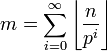so that pm divides n! but pm+1 does not.

(2) Based on this, the formula for R(p,n) is:which is equivalent to: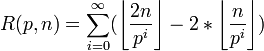(3) We can see that if pi is greater than 2n, then:

floor(2n/pi) = 0 and floor(n/pi) = 0 so that we have:

floor(2n/pi) - 2*(n/pi) = 0

so that we have:(4) Using the Division Algorithm (see Theorem 1, here), there exists q,r such that:

n = qpi + r

where r is less than pi

(5) From this, we can see that: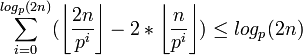since:

Case 1: r = 0

In this case, floor(2n/pi) = 2q and 2*floor(n/pi) = 2*q so that:

floor(2n/pi) - 2*floor(2n/pi) = 0

Case 2: r is less than (1/2)pi

In this case, floor(2n/pi) = 2q and 2*floor(n/pi) = 2*q so that:

floor(2n/pi) - 2*floor(2n/pi) = 0

Case 3: r ≥ (1/2)pi

In this case, floor(2n/pi) = 2q+1 and 2*floor(n/pi) = 2*q so that:

floor(2n/pi) - 2*floor(2n/pi) = 1

(5) So that we have:

pi ≤ plogp(2n) = 2n.

QED

Lemma 5:

For all i:

0 ≤ i ≤ 2n → (2n!)/(n!)(n!) ≥ (2n!)/(i!)(2n-i)!

Proof:

(1) We know that (see Definition 3, here for review of C(n,r) if needed):

C(2n,i-1) = (2n!)/(i-1)!(2n-i+1)!

C(2n,i) = (2n!)/(i!)(2n-i)!

(2) So, we see that:

C(2n,i) = (2n-i+1)/(i)*C(2n,i-1)

and

C(2n,i-1) = (i)/(2n-i+1)*C(2n,i)

(3) If i ≤ n, it follows that:

2n - i + 1 is greater than i since 2n - i + 1 ≥ n+1

So up to i=n, C(2n,i) is greater than C(2n,i-1)

(5) If i ≥ n+1, it follows that:

i is greater than 2n - i + 1 since 2n - i + 1 ≤ n

So down to i=n+1, C(2n,i-1) is greater than C(2n,i)

(6) So, we have shown that C(2n,n) is greater than C(2n,i) for i less than n and C(2n,n) is greater than C(2n,i) for i greater than n.

QED

Corollary 5.1: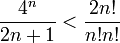Proof:

(1) Using the Binomial Theorem (see Theorem, here):(2) Using Lemma 5 above, we know that:(3) So that we have: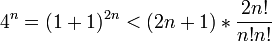(4) And dividing both sides by (2n+1) finishes the proof.

QED

Lemma 6:

If 2t is less than 8t, then t is less than 6

Proof:

(1) If t=6, then 2t is greater than 8t since:

26 = 64

8(6) = 48

(2) Assume it is true up to some t ≥ 6 so that: 2t is greater than 8t.

(3) Then 2*(2t)=2t+1 is greater than 2*8t = 16t

(4) 16t is greater than 8t+8 since 16=8t + 8t and for t ≥ 6, 8t is greater than 8.

(5) So that we have 2t+1 is greater than 8t+8 = 8(t+1).

QED

Lemma 7:

If:Then:

n is less than 2048.

Proof:

(1) Let n = 22t-1

(2) So 2n = 22t

(3) 22t = 2t

(4) So 2nln(2) = 2tln(2)

(5) (4)ln(2n) = 4ln(22t) = 2t*4*ln(2)

(6) Dividing both sides by ln(2) gives us:

2t is less than 8t

(7) Using Lemma 6 above, we conclude that t is less than 6.

(8) So, it follows that n is less than 22(6)-1 = 211 = 2048.

QED

Theorem: Bertrand's Postulate

For any n, there exists a prime p such that p is greater than n and less than 2n.

Proof:

(1) We know that Bertrand's Postulate is true for n less than 2048 [see Lemma 3 above]

(2) Assume for some n ≥ 2048, there is no prime between n and 2n.

(3) Let p be the largest prime factor of (2n!)/[(n!)(n!)] that is less than or equal to n.

(4) Using Lemma 1 above, we know that p ≤ 2n/3 since:

(a) Assume that that p is greater than 2n/3.

(b) Since n ≥ 2048, using Lemma 1 above, p is also greater than 2n

(c) This means that the highest power of x is given by (see Lemma 2 above):(d) Since 2n/3 is less than p, it follows that 2n is less than 3p, and further that 2n/p is less than 3 so that 2n/p ≤ 2.

(e) But from step #3 above, we know that n ≥ p so that we have n/p ≥ 1.

(f) This gives us that 2n/p ≤ 2 and n/p ≥ 1 so that we have:

x = floor(2n/p) - 2*floor(n/p) ≤ 2 - 2*1 = 0.

(g) So, under these circumstances, the highest power of x is 0 which means that there is no such p and we can conclude that p ≤ 2n/3.

(5) From step #4, we have:

(2n!)/(n!n!) = ∏ (p ≤ 2n/3) pR(p,n)

where R(p,n) is a function on p,n such that pR(p,n) divides (2n!)/(n!n!) but pR(p,n)+1 does not. [see Definition 1 above]

(6) From Lemma 2 above, we know that if p is greater than 2n, then R(p,n)=1 so that we have:(7) Since there are less than 2n primes less than 2n, using Lemma 4 above, we have:(8) If we use a limit for primorials (see Theorem, here), we have:(9) Using Corollary 5.1 above, we also have:(10) So, putting it all together, we have: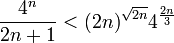(11) If we multiply 4(-2n/3) to both sides we get:(12) Now, since n ≥ 1, we note that 4n2 is greater than 2n+1

(a) For n=1, 4(1)2 is greater than 2(1)+1

(b) Assume that 4n2 is greater than 2n+1

(c) Since n ≥ 1, 4n2 + 8n is greater than 2n+1+2

(d) 4n2 + 8n + 1 is greater than 2(n+1) + 1

(e) And:

4(n+1)2 is greater than 2(n+1)+1

(13) So it follows that: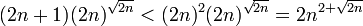(14) Since n is greater than 18, it follows that (4/3)√2n is greater than 2 + √2n since:

(a) 2(n) is greater than 6

(b) (4/3)√2n = (1/3)√2n + √2n

(c) And (1/3)√2n is greater than (1/3)*6 = 2.

(15) This gives us:(16) Putting it all together gives us: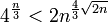(17) Taking the logarithm of both sides, gives us: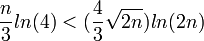(18) We can simplify this further by multiplying 3 to both sides and noting that 4=22 so that we get:(19) We can further simplify it by dividing both sides by 2n to get:(20) But now we use Lemma 7 above to reach a contradiction.

QED

References

### Primorial

The primorial is the product of primes less than or equal to a number n.

Definition: Primorial: n#

The primorial n# is defined as follows:Example:

1# = 1

3# = 3*(2#) = 3*2*(1#)=3*2

6# = 5# = 5*3*2

Theorem: if n≥ 1, then n# is less than 4n

Proof:

(1) It is true for (n=1 and n=2)

For n=1, 1#=1 is less than 4.

For n=2, 2#=2 is less than 42 = 16

(2) If n is even and n is greater than 2, then n is composite and we have:

n# = (n-1)# which is proven if we show that (n-1)# is less than 4n-1 since 4n-1 is less than 4n.

(3) To complete the proof, we show that n# is less than 4n if n is odd:

(a) By the inductive hypothesis, we can assume that it is true for all integers less than n and we assume that n is an odd integer where n=2x+1 and x ≥ 1 so that n ≥ 3.

(b) Now, we note that 4m = (1+1)2m = (2-1)*(1+1)2m+1 = (1/2)(1+1)2m+1

(c) Using the Binomial Theorem (see here), we note that:

(1+1)2m+1 = ∑ (i=0, 2m+1) (2m+1!)/[(i!)(2m+1-i)!]

(d) So,

(1/2)(1+1)2m+1 = (1/2) ∑ (i=0, 2m+1) (2m+1)!/[(i!)(2m+1-i)!]

is greater than:

(1/2) [(2m+1)!/(m!)(m+1)! + (2m+1)!/(m+1)!(m)!] = (2m+1)!/(m!)(m+1)!

(e) So, from (b) and (d), we can conclude that:

4m is greater than (2m+1)!/(m!)(m+1)!

since 4m = (1/2)(1+1)2m+1 which is greater than (2m+1)!/(m!)(m+1)!

(f) Each prime p that is greater than m+1 and less than 2m+1 divides (2m+1)!/(m+1)!(m!) since:

(2m+1)!/(m+1)!(m!) is an integer. [see Lemma 5, here]

Let u = (2m+1)!/(m+1)!(m!)

(2m+1)! = u(m+1)!(m!)

Since p does not divide (m+1)! and does not divide (m!), by Euclid's Generalized Lemma (see Corollary 2.1, here), p must divide u.

(g) This gives us:From the definition of primorial [see Definition above], step #3f above, and step #3e above.

(h) From the inductive hypothesis in step #3a, we can assume that:

(m+1)# is less than 4m+1

(i) So, we have (using step #3g above):

n# = (2m+1)# which is less (m+1)#*4m

(j) Using step #3h above, we have:

(2m+1)# is less than 4m+1*4m = 42m+1

QED

References

### Pascal's Rule

Theorem: Pascal's Rule

C(n,k) + C(n,k-1) = C(n+1,k)

where: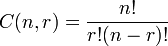Proof:

(1) We can find a common denominator for C(n,k) and C(n,k-1) so that we have: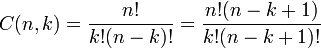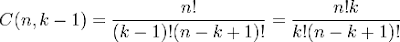(2) So that:(3) To complete the proof, we note that: n-k+1 = n+1-k so that we have:= C(n,k+1)

QED

References

## Monday, November 16, 2009

### Multiplicity of a Prime Factor

Definition 1: Multiplicity of a prime factor

The multiplicity of a prime factor of a given integer is the highest power of that factor that divides the integer.

Example 1: 60

The prime factorization of is 2*2*3*5

The multiplicity of 3 is 1. The multiplicity of 5 is 1. The multiplicity of 2 is 2.

Adrien-Marie Legendre came up with the following theorem regarding the multiplicity of a prime for n!. (For review of factorials (n!), see here)

Theorem 1: The multiplicity of a given prime p in n! is:That is, let x = the multiplicity of a prime p.

Then px divides n! but px+1 does not.

Proof:

(1) If p is greater than n, then p doesn't divide n! and the answer is 0.

(2) So, we can assume p ≤ n.

(3) Let k be the largest power of p such that pk ≤ n.

(4) For each 1 ≤ i ≤ k, the number of integers from 1 to n that are divisible by pi but not divisible by pi+1 is:If no numbers are divisible by pi, then floor(n/pi) = 0 [as does floor(n/pi+1)].

floor(n/pi+1) equals the number of integers that are greater than pi+1 and divisible by pi+1.

So the number of integers that are uniquely divisible by pi is the difference of these two floor functions.

(5) The value of px which divides n! is equal to the product of all the powers of p that divide any of the integers in the sequence from 1 .. n.

(6) So, the we can compute the value of x with: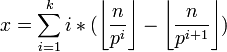(7) Putting this together, we see the following pattern: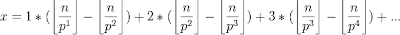(8) We can see for i=1, we have floor(n/p1), for i=2, we have 2*floor(n/p2) - 1*floor(n/p2), and for i=3, we have 3*floor(n/p3) - 2*floor(n/p3) and so on.

(9) The net result of this is that we rewrite the equation in step #7 to the following:QED

References:

## Monday, October 05, 2009

### Common roots with an irreducible polynomial

The content in today's blog is taken straight from Jean-Pierre Tignol's Galois Theory of Algebraic Equations.

Theorem:

Let f(x) be a rational function in one indeterminate over a field F.

Let V be a root of an irreducible polynomial R(x) ∈ F[X]

If f(V) = 0, then all roots of P are also roots of f.

Proof:

(1) Let f = P/Q where P,Q are polynomials and ∈ F[X] and Q(V) ≠ 0 and P(V) = 0.

(2) Since V is a root an irreducible polynomial R, it follows that R divides P. [see Lemma 2, here]

(3) Let W be any root of R.

(4) It follows that W is also a root of P since:

(a) If W is a root a R, then x - W divides R.

(b) Then it follows that x - W must also divide P since R divides P.

(4) It also follows that if W is a root of R, then W is not a root of Q since:

(a) Assume that W is a root of Q so that Q(W)=0

(b) Then it would follow that R divides Q.

(c) But then Q(V)=0 since R(V)=0

(d) But this is not true since Q(V) ≠ 0.

(e) Therefore, we reject our assumption in step #4a.

(5) Therefore, we have shown that P(W)=0 and Q(W) ≠ 0

(6) Hence f(W)=0 for any root W of the irreducible polynomial R.

QED

References

## Saturday, October 03, 2009

### A polynomial invariant on all but one variable

The content in today's blog is taken from Jean-Pierre Tignol's Galois' Theory of Algebraic Equations.

Lemma:

Let g be a polynomial in n indeterminates x1, ..., xn over some field K.

Let g be invariant under every permutation of x2, ..., xn

Then:

g can be written as a polynomial in x1 and the elementary symmetric polynomials s1, ..., sn-1 in x1, ..., xn

Proof:

(1) We can view g as a polynomial in x2, ..., xn with coefficients in K[x1].

(2) Using Waring's Method [see Theorem 4, here], we know that g can be written as a polynomial in the elementary symmetric polynomials s'1, ..., s'n-1 in x2, ..., xn with coefficients in K[x1]

(3) Therefore, there exists a polynomial g' such that:

g(x1, ..., xn) = g'(x1, s'1, ..., s'n-1)

where:

s'1 = x2 + ... + xn

s'2 = x2x3 + ... + xn-1xn

...

s'n-1 = x2*...*xn

(4) To complete the proof, we need to show that s'1, s'2, ..., s'n-1 can be restated in terms of s1, s2, ..., sn where:

s1 = x1 + ... + xn

s2 = x1x2 + ... + xn-1xn

...

sn = x1*...*xn

(5) We know that for any given polynomial [see Theorem 1, here]:

(X - x1)*...*(X - xn) = Xn - s1Xn-1 + ... + (-1)nsn

(6) Now, we can use the same principle to get:

(X - x2)*...*(X - xn) = Xn-1 - s'1Xn-2 + ... + (-1)n-1s'n-1

(7) Multiplying the above equation by (X - x1) gives us:

(X - x1)*...*(X - xn) = (X - x1)Xn-1 - (X - x1)s'1Xn-2 + ... + (X - x1)(-1)n-1s'n-1 =

Xn - (x1+s'1)Xn-1 + (x1s'1 + s'2)Xn-2 - (x1s'2 + s'3)Xn-3 + ... + (-1)n(x1s'n-1)

(8) Combining step #5 and step #7 gives us:

s1 = x1 + s'1

so that:

s'1 = s1 - x1

s2 = x1s'1 + s'2

so that:

s'2 = s2 - x1s'1 = s2 - x1(s1 - x1) = s2 - x1s1 + x12

and so on...

(9) Since we can subtitute all values s'i in terms of x1 and s1, ..., sn, we can use the equation in step #3 to get:

g(x1, ..., xn) = g'(x1, s'1, ..., s'n-1) = g'(x1, s1 - x1, s2 - s1x1 + x12, ... )

QED

References

## Thursday, October 01, 2009

### Nonzero Polynomials with Distinct Parameters

The following is taken from Harold M. Edwards in his book Galois Theory.

Theorem:

Let K be a field.

Let x1, x2, x3, ... be an infinite sequence of distinct elements of K

Let f(A,B,C,...) be a nonzero polynomial in n variables A,B,C,... with coefficients in K

Then:

It is possible to select values A=xj, B = xk, C = xm for the variables A,B,C from the sequence x1, x2, x3, ... so that F( xj, xk, xm, ...) ≠ 0

Proof:

(1) Assume that f(x) is a nonzero polynomial of one variable with degree m.

(2) Using the Fundamental Theorem of Algebra (see Theorem, here), we know that f(x) has at most m distinct roots.

(3) If we list off m+1 distinct elements of K from the infinite sequence, it is clear that at least one (let us say xr) will not be a root.

(4) So that f(xr) ≠ 0

(5) Assume that this is true up to n-1 variables for F(A,B,C...,Y) so that we know that F(xi, xj, ..., xy) ≠ 0

(6) Let G be a function of n variables so that we have G(A,B,C,...Z)

(7) Let H be a function on the first n-1 variables so that we have H(A,B,C,...Y) = G(A,B,C,...,Y,1)

(8) By assumption, we can find xi, xj, ... xy such that:

H(xi, xj, ..., xy) ≠ 0

(9) But then G(xi, xj, ..., xy, 1) ≠ 0.

QED

References
• Harold M. Edwards, Galois Theory, Springer, 1984

### Products of Nonzero Polynomials

The following is taken from Harold M. Edwards in his book Galois Theory.

Theorem: The product of nonzero polynomials is a nonzero polynomial

Proof:

(1) This theorem is clearly true in the case of one nonzero polynomial.

(2) Let's assume that it is true up to p-1.

(3) So that the product of p-1 nonzero polynomails is a nonzero polynomial g(x) of degree n so that we have:

g(x) = a0xn + a1xn-1 + ... + an-1x + an

where a0 is nonzero.

(4) Let us assume that f(x) is a nonzero polynomial of degree m so that:

f(x) = b0xm + b1xm-1 + ... + bm-1x + bm

where b0 is nonzero

(5) f(x)*g(x) is nonzero since:

the only term with degree m+n is a0*b0 which cannot be 0.

QED

References
• Harold M. Edwards, Galois Theory, Springer, 1984

## Tuesday, September 29, 2009

### The Discriminant

The content in today's blog is taken from Jean-Pierre Tignol's Galois' Theory of Algebraic Equations.

For a definition of symmetric polynomials, see Definition 1, here.

Lemma 1:

LetThen:

Δ(x1, ..., xn)2 is a symmetric polynomial

Proof:

(1) Let P = ∏ (xi - xj)

(2) If any xi = xj, then P = 0. [that is, if there is a multiple root]

(3) Assume that there are no multiple roots.

(4) If we swap any two roots, then the result is either P or -P, then the result is to permute the ordering of each of the differences and to change the signs of some.

(5) Ordering doesn't change the product so the only the change that occurs is the sign of the product. That is, the result is P or -P depending upon which parameters get swapped.

(6) So it is clear that ∏ (xi - xj) is not symmetric.

(7) If we permutate the values of [∏ (xi - xj)]2, it is clear that the result is always P2 = P2 = (-P)2

QED

Using Waring's Method (see Theorem 4, here), we know that [∏ (xi - xj)]2 can be expressed as a function of the elementary symmetric polynomials (for review, see here) so that we have:

Definition 1: The Discriminant Δ

LetThen the Discriminant D is:

D(s1, ..., sn) = Δ(x1, ..., xn)2

where s1, ..., sn are the elementary symmetric polynomials.

Example 1: Discriminant of a generic polynomial of degree 2

D(s1,s2) = s12 - 4s2

First, we carry out the multiplication:

Δ(x1,x2)2 = (x1 - x2)2 = x12 + x22 - 2x1x2

Then, we show it as a function of the elementary symmetric polynomials:

x12 + x22 - 2x1x2 = (x1 + x2)2 - 4x1x2=s12 - 4s2

Example 2: Discriminant of a generic polynomial of degree 3

D(s1,s2,s3) = s12s22 + 18s1s2s3 - 27s32 -4s13s3 - 4s23

We note that:

Δ(x1,x2,x3) = (x1 - x2)(x1 - x3)(x2 - x3)

We can simplify this by restating Δ(x1,x2,x3) as:

Δ(x1,x2,x3) = A - B

where:

A = x12x2 + x22x3 + x32x1

and

B = x1x22 + x2x32 + x3x12

So that:

Δ(x1,x2,x3)2 = (A - B)2 = A2 + B2 - 2AB = (A + B)2 - 4AB

Now, we note that A+B and AB are symmetric polynomials and using Waring's method (see Theorem 4, here), we have:

A + B = ∑ x12x2 = s1s2 - 3s3

AB = ∑ x14x2x3 + ∑ x13x23 + 3x12x22x32 = s13s3 + s23 - 6s1s2s3 + 9s32

Now, we can combine these results to get the discriminant:

(A + B)2 - 4AB = ( s1s2 - 3s3)2 - 4( s13s3 + s23 - 6s1s2s3 + 9s32) =

= s12s22 + 18s1s2s3 - 27s32 -4s13s3 - 4s23

Example 3: Discriminant of x3 + px + q

D(s1,s2,s3) = -27q2 - 4p3

First, we note that the values of the elementary symmetric polynomials can be derived from the coefficients of a polynomial (see Theorem 1, here) so that:

s1 = 0

s2 = p

s3 = -q

So that:

s12s22 + 18s1s2s3 - 27s32 -4s13s3 - 4s23= 0 + 0 - 27(-q)2 - 0 - 4p3 = -27q2 - 4p3

Theorem 2:

Let P ∈ R[X] be a monic polynomial with real coefficients, which splits into a product of linear factors over C such that:

P = (x - u1)*...*(x - un)

for some u1, ..., un ∈ C.

Let d ∈ R be the discriminant of P

The equality d=0 holds if and only if P has a root of multiplicity at least 2 in C

If all the roots of P are real, then d ≥ 0. If n=2 or n=3 and not all the roots are real, then d ≤ 0.

Proof:

(1) d = ∏ (ui - uj)2 where 1 ≤ i is less than j ≤ n

(2) If P has a root of multiplicity at least 2, then d = 0 since we have a case where ui = uj

(3) If all the roots are real, then d ≥ 0 since any real number squared is greater or equal to 0 and product of nonnegative numbers is greater or equal to 0.

(4) Assume n =2

(5) d = (u1 - u2)2 [see Definition 1 above]

(6) If u1 is not real, then u2 = u1 [see Theorem 5, here]

(7) Let u1 = a + bi

(8) Let u2 = a - bi

(9) (u1 - u2)2 = (a + bi - [a - bi])2 = (2bi)2 = -4b2 = -abs(4*b2)

(10) So that d ≤ 0.

(11) Assume that n = 3

(12) Then, d = (u1 - u2)2(u1 - u3)2(u2 - u3)2

(13) Assume that not all three roots are real. So, we can assume that u1 is not real.

(14) Then, it follows that its conjugate is also a root. So we can assume that u2 is not real and u1 = u2

(15) We know that u3 is then real. [see Theorem 3, here]

(16) So there exists real numbers a,b,c such that:

u1 = a + bi

u2 = a - bi

u3 = c

And we have:

(u1 - u2)(u1-u3)(u2 - u3) = [a+bi - (a - bi)][a+bi - c][a-bi - c] = (2bi)([a-c]+bi)([a-c]-bi)

Now, we know that:

([a - c] + bi)([a - c] - bi) = [a - c][a - c] - bi[a - c] + bi[a - c] - [bi][bi] =[a - c]2 + b2

Combining this with the above we get:

(2bi)[(a - c)2 + b2] = i[(2b)(a - c)2 + 2b2]

Now, it is clear that (2b)(a - c)2 + 2b2 is a real number since a,b,c are real and we can set s = (2b)(a - c)2 + 2b2 where s is a real number.

So d = (is)2 = -(s2) = -abs(s2)

(17) So, d ≤ 0.

QED

Corollary 2.1:

x3 + px +q = 0

has three distinct real solutions if and only if (p/3)3 + (q/2)2 is less than 0.

Proof:

(1) By Exercise 3 above, the discriminant of x3 + px +q is d = -27q2 - 4p3

We further note that:

d = -27q2 - 4p3 = -2233[(p/3)3 + (q/2)2]

(2) Now if (p/3)3 + (q/2)2 is less than 0, it follows that d ≥ 0.

(3) So, using Theorem 2 above, we are done.

QED

References